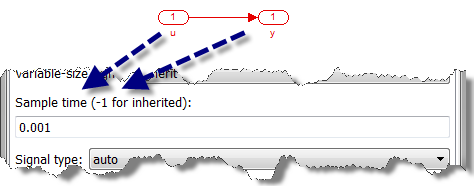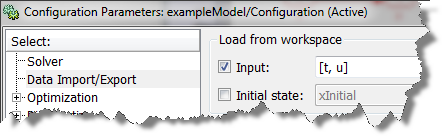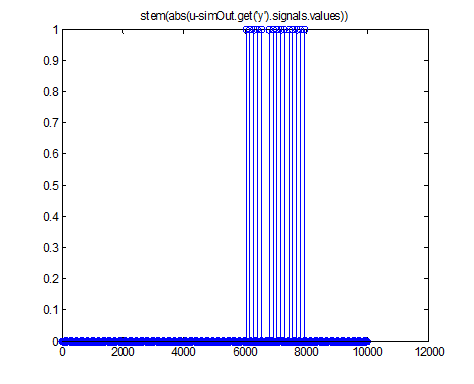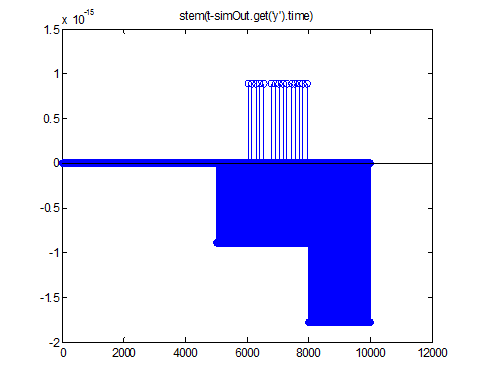August 2019 Update: See blog post Loading Signals in Timetable Format for an improved way to address the issue described in this post.

-----------------

Today I want to look at a problem that often frustrates Simulink users who have discrete inputs to their model.

The Problem

I create a simple model with just an Inport block connected directly to an Outport block, both configured to execute at a sample time of 0.001 second.I configure the model to import data from the workspace:I simulate the model and compare the input with the output using this code:

ts = 0.001; t = (0:ts:10)'; u = (1:length(t))'; simOut = sim('exampleModel'); stem(abs(u-simOut.get('y').signals.values))

For some samples, the output does not match the input.The explanation

To understand what is happening here, it is important to compare the time vector of the input data and the time vector used by Simulink.As we can see in this image, the steps taken by the Simulink solver are different from the ones specified in the input time vector. As explained in technical solution 1-1ITHX9, Simulink computes time using the following equation:

 time = stepSize * [0:N]' 

While these two methods look similar, they can give different results due to floating point round-off. As you can image, if the input data is slightly behind or before the step taken by the solver, a data point can be skipped or repeated.

The Solution

To avoid such problem, it is recommended to NOT provide the time vector when inputting discrete data into a simulation. For example, modifying the code to use a structure without time will allow the Inport to take the next sample from the vector at each step. Here is some code to create a signal structure without time, update the model to use this structure, and verify that the input and output are equal.

inputStruct.time = []; inputStruct.signals.dimensions = 1; inputStruct.signals.values = u; set_param('exampleModel','ExternalInput','inputStruct') simOut = sim('exampleModel'); norm(u-simOut.get('y').signals.values) ans = 0